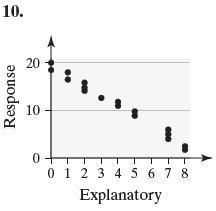×
Get Full Access to Statistics: Informed Decisions Using Data - 5 Edition - Chapter 4.1 - Problem 10
Get Full Access to Statistics: Informed Decisions Using Data - 5 Edition - Chapter 4.1 - Problem 10

×

# ?In Problems 9–12, determine whether the scatter diagram indicates that a linear relation may exist between the two variables. If the relation is lineaISBN: 9780134133539 240

## Solution for problem 10 Chapter 4.1

Statistics: Informed Decisions Using Data | 5th Edition

• Textbook Solutions
• 2901 Step-by-step solutions solved by professors and subject experts
• Get 24/7 help from StudySoup virtual teaching assistantsStatistics: Informed Decisions Using Data | 5th Edition

4 5 1 328 Reviews
29
1
Problem 10

In Problems 9–12, determine whether the scatter diagram indicates that a linear relation may exist between the two variables. If the relation is linear, determine whether it indicates a positive or negative association between the variables.Step-by-Step Solution:

Step 1 of 5) In Problems 9–12, determine whether the scatter diagram indicates that a linear relationship may exist between the two variables. If the relation is linear, determine whether it indicates a positive or negative association between the variables. Generally, there should be between 5 and 20 classes. The smaller the data set, the fewer classes you should have. For example, we might choose 12 classes for the data in Table 12. r Determine the class width by computing Class width ≈ largest data value - smallest data value number of classes Round this value up to a convenient number. For example, using the data in Table 12, we obtain class width ≈ 19.43 - 8.28 12 = 0.929. We round this up to 1 because this is an easy number to work with. Rounding up may result in fewer classes than were originally intended.

Step 2 of 2

## Discover and learn what students are asking

Calculus: Early Transcendental Functions : Functions of Several Variables
?In Exercises 15 - 22, find all first partial derivatives. $$f(x, y)=e^{x} \cos y$$

Calculus: Early Transcendental Functions : Parametric Equations and Calculus
?In Exercises 1-4, find $$d y / d x$$. $$x=2 e^{\theta}, y=e^{-\theta / 2}$$

Calculus: Early Transcendental Functions : Second-Order Nonhomogeneous Linear Equations
?Verifying a Solution In Exercises 1-4,verify the solution of the differential equation. Solution

Statistics: Informed Decisions Using Data : Tests for Independence and the Homogeneity of Proportions
?Social Well-Being and Obesity The Gallup Organization conducted a survey in 2014 asking individuals questions pertaining to social well-being such as

Unlock Textbook Solution